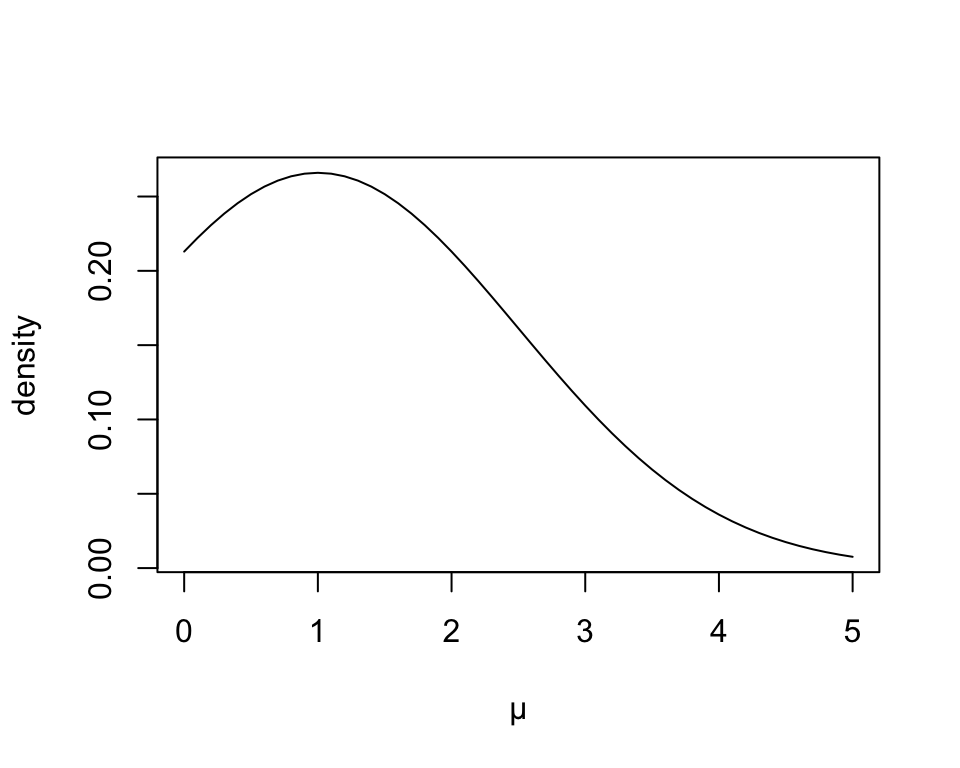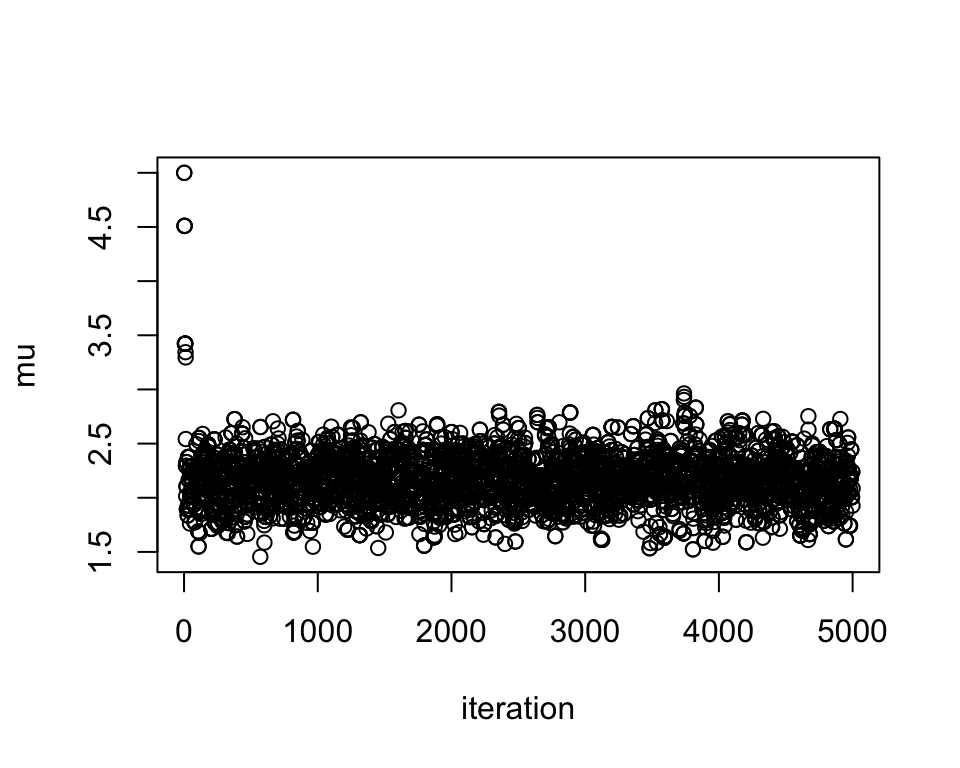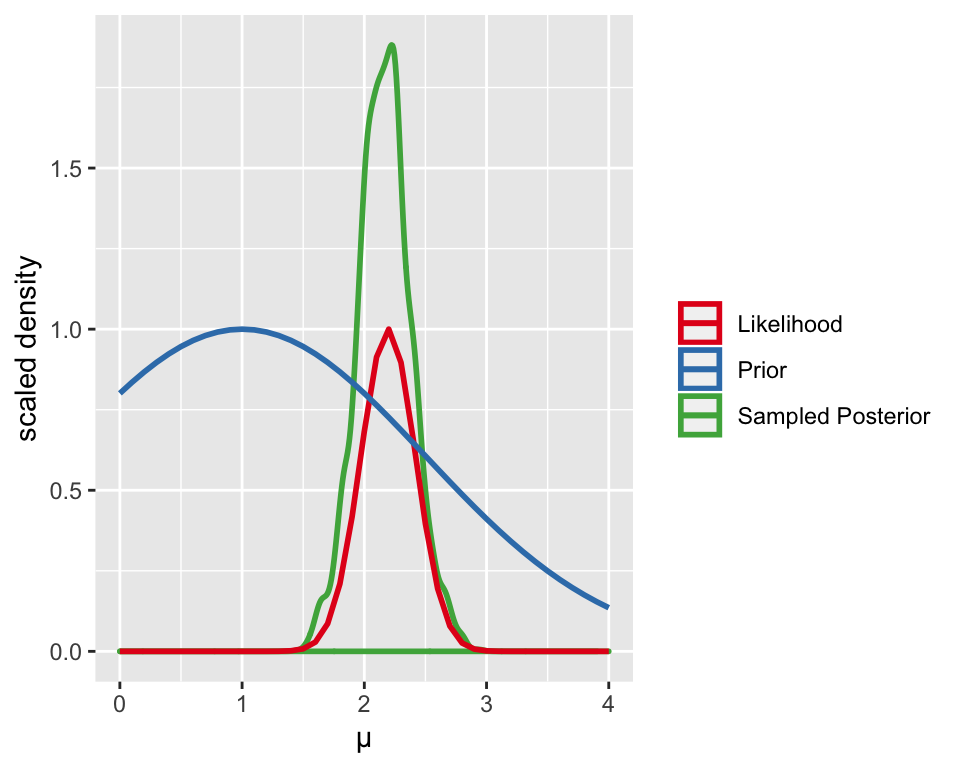### Setup

library(ggplot2)
set.seed(2)

### Model, Data, & Likelihood

Let’s build a simple MCMC sampler using the Metropolis algorithm. First, we’ll set up our model. We’ll suppose the data ($$z$$) comes from a normal distribution, with a known standard deviation $$\sigma = 1$$: $z \sim \mathcal{N}(\mu, 1)$ Then our the likelihood for a single data point $$z_i$$ is given by: $P(z_i \,|\, \mu, 1) = f(z_i \,|\, \mu, 1) = \frac{1}{\sqrt{2\pi}}e^{-\frac{(z - \mu)^2}{2}}$ and our overall likelihood for a full data set $$z = \{z_1,\dots, z_n\}$$ is given by: $P(z \,|\, \mu) = \prod_{i=1}^n f(z_i \,|\, \mu, 1)$

likelihood = function(data,mu){prod(dnorm(data,mu,1))}

From the above model, let’s simulate a little data set that we can use for parameter estimation:

data = rnorm(20,2,1)

### Prior Distribution

Now, we need a prior for our unknown parameter $$\mu$$. We’ll choose a normal distribution for the prior of $$\mu$$. But you can also try other priors (e.g. uniform) too! For our prior $$P(\mu)$$, we’ll choose $$\mu \sim \mathcal{N}(1,1.5)$$.

# Set up prior
prior = function(mu){dnorm(mu,1,1.5)}

# Plot the prior
plot(seq(0,5,0.1),prior(seq(0,5,0.1)),type='l',xlab="µ", ylab="density")### Setting up the Metropolis algorithm for MCMC

As we saw in class, the Metropolis algorithm for MCMC is:

1. Initialization
• Choose a starting point in parameter space, say $$\mu = 5$$.
• Decide on a proposal distribution to choose the next point to sample—this distribution is often somewhat arbitrary, but different choices will make it easier or harder to converge to the stationary distribution. In our case we’ll choose a normal distribution with mean given by the current sampled parameter value (call this $$\mu_{curr}$$), and standard deviation of 0.5, $$\mathcal{N}(\mu_{curr}, 0.5)$$.
• Decide how many samples you want to take—in our case, let’s take 5000.

We’ll also make a variable, samples, to hold our MCMC samples as we go.

mu.curr = 5 #start value for mu
proposaldist = function(paramval){rnorm(1,paramval,0.5)}
numsteps = 5000
samples = numeric(numsteps)
1. For each iteration,
• Use the proposal distribution to draw a new proposed sample, $$\mu_{next}$$

• Calculate the product of the likelihood and the prior, $$P(z \,|\, \mu) \cdot P(\mu)$$, for both the current and newly proposed values of $$\mu$$. Note that $$P(z \,|\, \mu) \cdot P(\mu)$$ is proportional to the posterior (it is just missing the denominator from Bayes’ theorem), so our sampler will recover the posterior distribution.

• Calculate the acceptance ratio, $$a = \frac{P(z \,|\, \mu_{next}) \cdot P(\mu_{next})}{P(z \,|\, \mu_{curr}) \cdot P(\mu_{curr})}$$.

• If $$a\geq 1$$, the new point is as good or better than the last, and we automatically accept.

• If $$a<1$$, then the new point $$\mu_{next}$$ is worse. In this case, we accept the new point with probability $$a$$. If it is accepted, we set mu.curr = mu.next. If it is not accepted, we leave mu.curr as is and re-draw mu.next in the next iteration.

• Also, don’t forget to record your mu.curr samples at each iteration!

Code the algorithm above and generate 5000 samples for $$\mu$$. Here’s more explicit pseudocode for 2) to help you set things up:

• For each iteration:
• Set samples[i] = mu.curr (i.e. record the current value of $$\mu$$)
• Draw mu.next from proposaldist, using mu.curr
• Calculate $$likelihood*prior$$ for mu.curr, e.g probcurr = likelihood(data,mu.curr)*prior(mu.curr)
• Calculate $$likelihood*prior$$ for mu.next (let’s call this probnext)
• Set acceptratio equal to probnext/probcurr
• If acceptratio >= 1, accept mu.next—in other words, set mu.curr = mu.next
• If acceptratio < 1, then accept mu.next with probability acceptratio (i.e. if runif(1)<acceptratio)

### Plotting the Results

Next, plot the chain—do you see burn-in? Roughly when does it converge to the stationary distribution?

plot(1:numsteps,samples, xlab='iteration',ylab='mu')Lastly, if we plot the prior, likelihood, and posterior, we can see how the likelihood alters the prior to generate the posterior. We can also compare the sampled posterior matches the analytical posterior.

# take just the later part of the chain, after burn-in
posteriorsample = samples[500:numsteps]

# set up the prior & likelihood for line-plotting
# (also re-scale them so they're on similar y-axis scales)

paramvalslist = seq(0,4,0.1) # parameter values list

#corresponding prior values
priorlist = prior(paramvalslist)
priorlist = priorlist/max(priorlist)

#likelihood values
likelihoodlist = numeric(length(paramvalslist))
for(i in 1:length(paramvalslist)){likelihoodlist[i] = likelihood(data,paramvalslist[i])}
likelihoodlist = likelihoodlist/max(likelihoodlist)

# This is the dataframe we'll use for ggplot
plotdata = data.frame(paramvalslist,priorlist,likelihoodlist)

ggplot(plotdata) +
geom_density(data=as.data.frame(posteriorsample),aes(posteriorsample, color='Sampled Posterior'),size=1) +
geom_line(aes(paramvalslist,likelihoodlist, color='Likelihood'),size=1) +
geom_line(aes(paramvalslist,priorlist, color='Prior'),size=1) +
labs(x="µ", y="scaled density") + guides(color = guide_legend('')) +
scale_colour_brewer(palette = "Set1")### Extra problems

• Try changing the standard deviation in the proposal distribution—how does it change the behavior of the chain? Try this for different numbers of iterations too—sometimes it’s easier to see the differences with fewer iterations (e.g. 500 or 1000).

• Try changing your prior to something else, e.g. uniform on $$(0,5)$$. How does that change things? Try other priors too!

• Try changing your data set to add more or fewer data points—how does that change the convergence of your chain?

• Feeling ambitious and computational? Try coding up a 2-D sampler, and estimate both $$\mu$$ and $$\sigma$$. If you do, try ploting your chain as it moves around in your 2D parameter space, and you can even show the posterior as a contour plot and see how it’s moving up and down the distribution!

• Feeling ambitious and mathy? The normal distribution is the conjugate prior for a normal likelihood—that means that for our model and prior, we should be able to derive a closed form normal posterior for $$\mu$$. Derive it, and plot it with your sampled posterior. How well do they match? How does this change as you change the number of iterations you run?